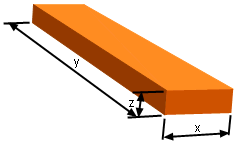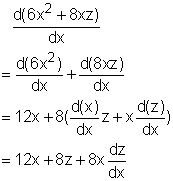Ch 2. Derivatives I Multimedia Engineering Math DerivativeFormulas TrigonometricFunctions Differentiability ChainRule ImplicitDifferentiation
 Chapter 1. Limits 2. Derivatives I 3. Derivatives II 4. Mean Value 5. Curve Sketching 6. Integrals 7. Inverse Functions 8. Integration Tech. 9. Integrate App. 10. Parametric Eqs. 11. Polar Coord. 12. Series Appendix Basic Math Units Search eBooks Dynamics Fluids Math Mechanics Statics Thermodynamics Author(s): Hengzhong Wen Chean Chin Ngo Meirong Huang Kurt Gramoll ©Kurt GramollMATHEMATICS - CASE STUDY SOLUTIONRectangular Box Recall Tony made a rectangular box with 100 in2 surface area in his arts and crafts class. The length of his box is 3 times of its width. He wondered how the height changes affects width's enlargement? Let the length of the box be y, the width be x and the height be z. Let the surface area of the box be s. The surface area of the box can be calculated as      s = 2xy + 2xz + 2yz Since the length is 3 times of the box's width, substitute y = 3x into the equation.      s = 2x(3x) + 2xz + 2z(3x)         = 6x2 + 2xz + 6zx         = 6x2 + 8xz Because the height z is implicitly defined by its width x, the expression, the height z changes with respect to its width x (dz/dz), can be calculated using implicit differentiation method. Differentiate both side of surface area equation with respect to x      ds/dx = d(6x2 + 8xz)/dx The left hand side of the equation, ds/dx is zero since the surface area is constant at 100 in2. The right hand side isThe left hand side equals the right hand side, so      12x + 8z + 8xdz/dx = 0 Rearranging gives      dz/dx = -(3x + 2z)/2x = -1.5 + z/x